# Analytic geometry + Pythagorean theorem - math problems

#### Number of problems found: 65

• DistanceWhat is the distance between the origin and the point (18; 22)?
• Segment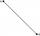Calculate the segment AB's length if the coordinates of the end vertices are A[10, -4] and B[5, 5].
• Distance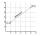Calculate distance between two points X[18; 19] and W[20; 3].4 m long ladder touches the cube 1mx1m at the wall. How high reach on the wall?
• Distance problemA=(x, x) B=(1,4) Distance AB=√5, find x;
• Calculate 8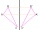Calculate the coordinates of point B axially symmetrical with point A[-1, -3] along a straight line p : x + y - 2 = 0.
• CenterCalculate the coordinates of the circle center: x2 -4x + y2 +10y +25 = 0
• Circle - AGFind the coordinates of circle and its diameter if its equation is: ?
• Distance problem 2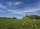A=(x,2x) B=(2x,1) Distance AB=√2, find value of x
• Find parametersFind parameters of the circle in the plane - coordinates of center and radius: ?
• Distance between 2 pointsFind the distance between the points (7, -9), (-1, -9)
• CircleWrite the equation of a circle that passes through the point [0,6] and touch the X-axis point [5,0]: ?
• Angle of the body diagonalsUsing vector dot product calculate the angle of the body diagonals of the cube.
• Vertices of RTShow that the points P1 (5,0), P2 (2,1) & P3 (4,7) are the vertices of a right triangle.
• Square side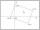Calculate length of side square ABCD with vertex A[0, 0] if diagonal BD lies on line p: -4x -5 =0.
• Angle between vectorsFind the angle between the given vectors to the nearest tenth of a degree. u = (-22, 11) and v = (16, 20)
• Triangle IRTIn isosceles right triangle ABC with right angle at vertex C is coordinates: A (-1, 2); C (-5, -2) Calculate the length of segment AB.
• Parametric formCalculate the distance of point A [2,1] from the line p: X = -1 + 3 t Y = 5-4 t Line p has a parametric form of the line equation. ..
• Vertex pointsGiven the following points of a triangle: P(-12,6), Q(4,0), R(-8,-6). Graph the triangle. Find the triangle area.
• Find the 3Find the distance and midpoint between A(1,2) and B(5,5).

Do you have an interesting mathematical word problem that you can't solve it? Submit a math problem, and we can try to solve it.

We will send a solution to your e-mail address. Solved examples are also published here. Please enter the e-mail correctly and check whether you don't have a full mailbox.

Please do not submit problems from current active competitions such as Mathematical Olympiad, correspondence seminars etc...

Line slope calculator is helpful for basic calculations in analytic geometry. The coordinates of two points in the plane calculate slope, normal and parametric line equation(s), slope, directional angle, direction vector, the length of the segment, intersections of the coordinate axes, etc. Pythagorean theorem is the base for the right triangle calculator. Analytic geometry - math problems. Pythagorean theorem - math problems.